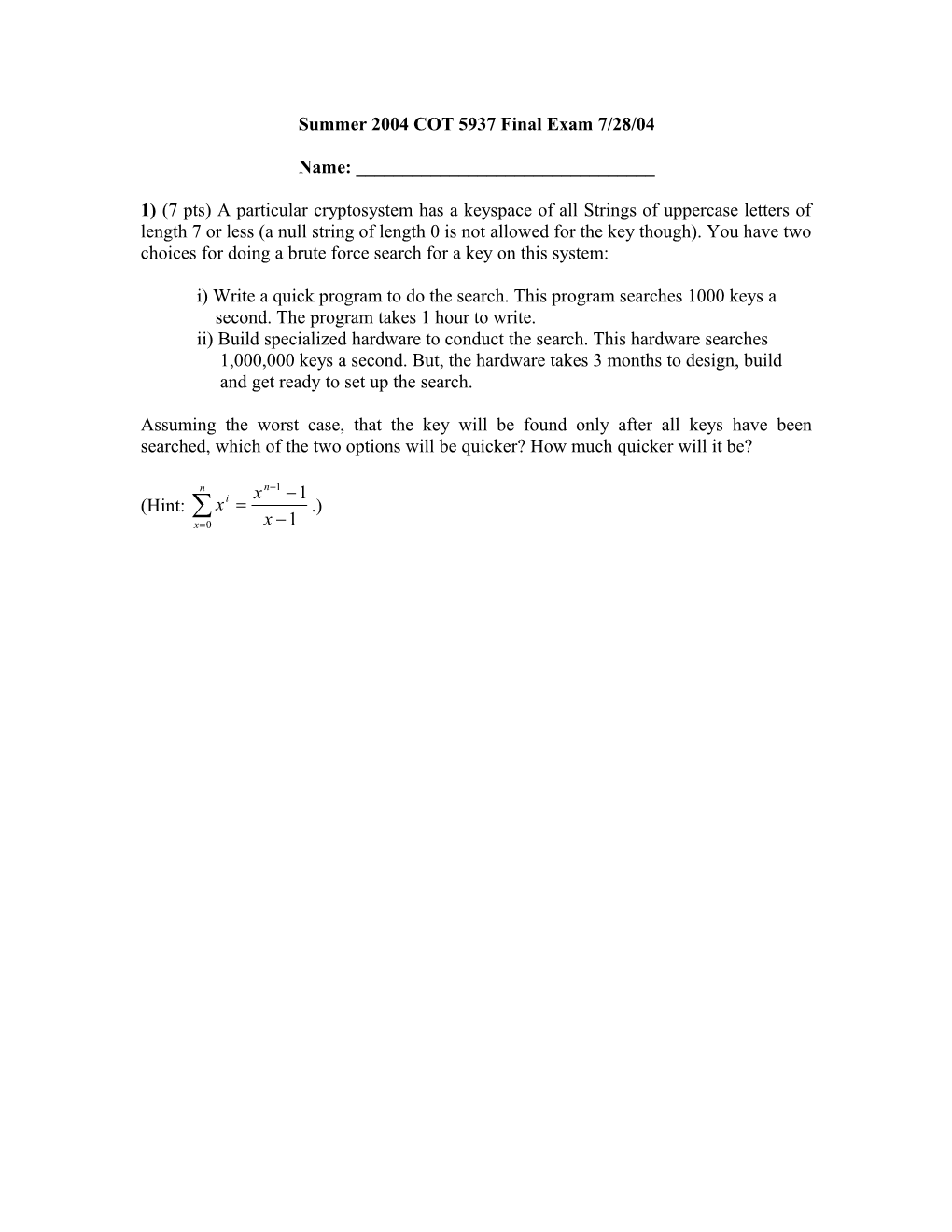# Summer 2004 COT 5937 Final Exam 7/28/04Summer 2004 COT 5937 Final Exam 7/28/04

Name: ______

1) (7 pts) A particular cryptosystem has a keyspace of all Strings of uppercase letters of length 7 or less (a null string of length 0 is not allowed for the key though). You have two choices for doing a brute force search for a key on this system:

i) Write a quick program to do the search. This program searches 1000 keys a

second. The program takes 1 hour to write.

ii) Build specialized hardware to conduct the search. This hardware searches

1,000,000 keys a second. But, the hardware takes 3 months to design, build

and get ready to set up the search.

Assuming the worst case, that the key will be found only after all keys have been searched, which of the two options will be quicker? How much quicker will it be?

(Hint: .)

2) (10 pts) The following ciphertext was obtain using the Hill cipher with the key . The alphabet size is 16, so A=0, B=1, ..., P=15 are the valid letters in the plain and ciphertexts. (Also, the message is processed in columns, so for example, BC would be encrypted as follows: , which is equivalent to NA. Find the corresponding decryption key and plaintext for the ciphertext message below:

PFBJAPJL

3) (10 pts) In a specific execution of DES on a single block, both the block of data to be encrypted and the key are bit strings of all 1's. (The block contains 64 1's and the key contains 56 1's.) In this situation, what is the value of R1, the right 32 bits of the output after the first round of DES? Please answer in hexadecimal.

4) (10 pts) In a specific execution of 128 bit AES, the message is

0123456789ABCDEFFEDCBA9876543210

and the key is

FFFFFFFFFFFFFFFFFFFFFFFFFFFFFFFF

What does the state matrix look like after the first execution of Shift Rows? Initially, the state matrix looks like this: .

5) (15 pts) Let p be an arbitrary odd prime, and g be a positive integer such that 1 < g < p. If for all positive integers a such that a|(p-1) we find that ga 1 (mod p), prove that g is a primitive root mod p.

6) (5 pts) Using the result proven in question 6, prove that 5 is a primitive root mod 47. Show the relevant work and describe how you performed operations on your calculator.

7) (10 pts)Solve the following set of equations for an unique value mod 11550. (You may omit the work of finding inverses here if you wish. But if your answer is incorrect, that work could earn you partial credit.)

x  13 mod 21

x  3 mod 22

x  7 mod 25

8) (5 pts) Calculate 756001 mod 1025 as efficiently as possible.

9) (8 pts) In an RSA system, you are given that n = 209 and e = 59. Find d. (Show all relevant work, including the extended Euclidean algorithm.)

10) (10 pts) Consider the elliptic curve E43(2, 5). Let P = (5, 11) and Q = (12, 37). Calculate both 2P and P+Q.

11) (10 pts) Use the Fermat factorization method to factor 26500891, showing all of your work. (Write out all tests you run on the calculator. You can test to see if a number is a perfect square just by taking its square root and seeing if you get an integer.)

12) (10 pts bonus) Let S be a string of length n, indexed from 0 to n-1. Also, let n = ab = cd, where a, b, c, and d are all distinct integers greater than 1. (Thus, there are only some values of n that are permissible.) Define a double transpose as follows:

i) First copy S into a two-dimensional matrix with a rows and b columns, copying

in values in each row, left to right, then top to bottom.

ii) Transpose this matrix.

iii) Read the values in this current matrix, row by row, left to right, then top to

bottom back into S.

iv) Now copy this version of S into a two-dimensional matrix with c rows and d

columns, using the same method in step i.

v) Transpose this matrix.

vi) Read the values in this current matrix back into S as done in step iii.

This current state of S is the "double transpose" of it.

Here's a small example with S = "ABCDEFGHIJKL", a=3, b=4, c=2, d=6:

Initial matrix = Transposed matrix =

After step 3, S = "AEIBFJCGKDHL"

Step 4 matrix = Transposed matrix =

So, the final output value for S is "ACEGIKBDFHJL"

Prove or disprove: Regardless of the order of the two transposes in the double transpose, the final output of the double transpose is the exact same. (In our example, the other way we could have ordered our transposes is by using the 2x6 matrix first, followed by the 3x4 matrix.)